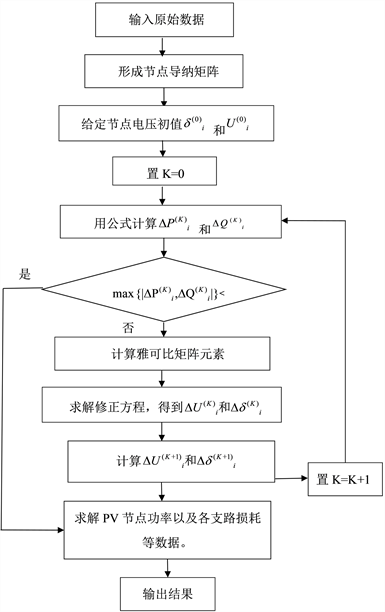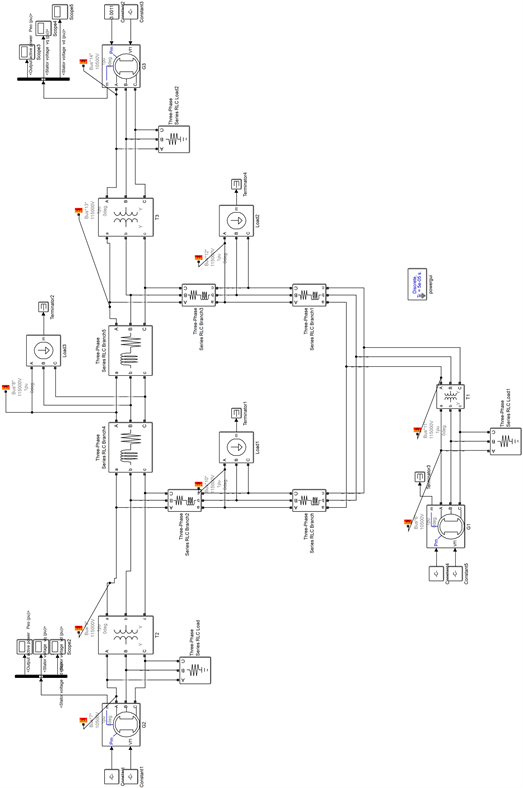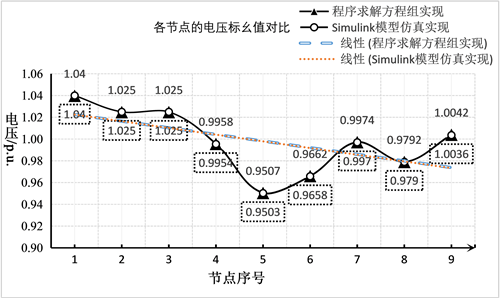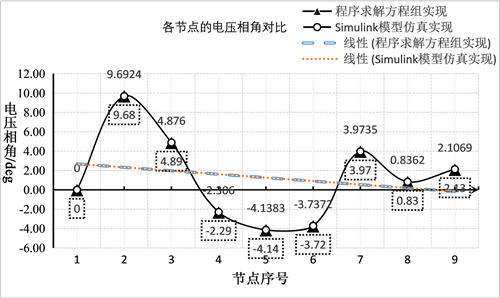﻿ 电力系统潮流分析的牛顿–拉夫逊法计算

电力系统潮流分析的牛顿–拉夫逊法计算Newton-Raphson Method for Power Flow Analysis of Power System

Abstract: Power flow calculation is mainly based on a given grid architecture, parameters and other condi-tions to determine the stable operation parameters of various components of a power system. By studying the principle of Newton-Raphson method, this paper introduces it into power flow calcula-tion and establishes the power system model and algorithm. Then, taking three machines and nine nodes as a specific example, using the Newton-Raphson method’s program to calculate, and then using the power system model in Simulink simulation to calculate. The results of the two methods are consistent, which shows that the Newton-Raphson method has good results in dealing with the power flow calculation.

1. 引言

2. 算法原理与实例分析

2.1. 一个三机九节点算例Figure 1. Schematic diagram of the structure of a 3-machine 9-node power systemTable 1. Generator data of 3-machine 9-node systemTable 2. Transmission line and transformer data of 3-machine 9-node systemTable 3. Load parameters of a 3-machine 9-node system

2.2. 牛顿–拉夫逊法潮流计算原理

$f\left({\chi }_{1},{\chi }_{2},\cdots ,{\chi }_{n}\right)=0\left(i=1,2,\cdots ,n\right)$ (1)

${\chi }^{\left(0\right)}$ 为初始估计值， $\chi$${\chi }^{\left(0\right)}$ 附近，利用泰勒级数展开并忽略高阶项后得：

$f\left({\chi }^{\left(0\right)}+\Delta {\chi }^{\left(0\right)}\right)=f\left({\chi }^{\left(0\right)}\right)+{f}^{\prime }\left({\chi }^{\left(0\right)}\right)\Delta {\chi }^{\left(0\right)}$ (2)

$\Delta {\chi }^{\left(0\right)}=-{\left[{f}^{\prime }\left({\chi }^{\left(0\right)}\right)\right]}^{-1}f\left({\chi }^{\left(0\right)}\right)$ (3)

(3)式为第一次迭代得出的修正量，将 $\Delta {\chi }^{\left(0\right)}$${\chi }^{\left(0\right)}$ 相加后得到变量的改进值 ${\chi }^{\left(1\right)}$，如(4)式：

${\chi }^{\left(1\right)}={\chi }^{\left(0\right)}+\Delta {\chi }^{\left(0\right)}$ (4)

${f}^{\prime }\left({\chi }^{\left(k\right)}\right)\Delta {\chi }^{\left(k\right)}=-f\left({\chi }^{\left(k\right)}\right)$ (5)

${\chi }^{\left(k+1\right)}={\chi }^{\left(k\right)}+\Delta {\chi }^{\left(k\right)}$ (6)

$\mathrm{max}\left\{|f\left({\chi }_{1}^{\left(\text{k}\right)},{\chi }_{2}^{\left(k\right)},\cdots ,{\chi }_{n}^{\left(k\right)}\right)|\right\}<{\epsilon }_{1}$ (7)

2.3. 牛顿–拉夫逊潮流算法流程

2.4. 电网的参数求解

${R}_{T}=\frac{{P}_{K}}{1000}\frac{{U}_{N}^{2}}{{S}_{N}^{2}}$${X}_{T}=\frac{{U}_{K}%}{100}\frac{{U}_{N}^{2}}{{S}_{N}}$${B}_{T}=\frac{{I}_{0}%}{100}\frac{{S}_{N}}{{U}_{N}^{2}}$${G}_{T}=\frac{{P}_{0}}{1000{U}_{N}^{2}}$ (8)Figure 2. Block diagram of power flow calculation program with Newton-Raphson method

2.5. 通过导纳矩阵计算出结果

3.1. 系统的搭建Figure 3. Simulation diagram of 3-machine 9-node system

3.2. 模块参数的计算及设置

1) 发电机模型参数设置

2) 变压器模型参数设置

3) 线路模型参数计算及设置

4) 负荷模型参数设置

3.3. 计算结果Figure 4. Comparison of voltage unit value of each nodeFigure 5. Voltage phase angle comparison of each node

3.4. 对比分析

2) 数据分析的差异。在同样的数据填入电力系统模型下，Matlab程序利用矩阵可以有选择性地不填入发电机、变压器电抗电阻等数据；而在Powergui模型中，则需要填入较为具体及详细的参数，模型较为复杂，但是模型的搭建更加直观、更贴合实际生活。

4. 总结

NOTES

*通讯作者。

 马为民, 吴方劼, 杨一鸣, 等. 柔性直流输电技术的现状及应用前景分析[J]. 高电压技术, 2014, 40(8): 2429-2439.

 瞿吉, 安允展, 董学育. 直流微电网协调控制策略研究[J]. 电工技术, 2018(24): 115-116.

 徐劲松, 宁玉琳, 杨永峰. 基于Matlab的电力系统PQ分解法潮流计算研究[J]. 电气传动自动化, 2011, 33(2): 10-16.

 张丽英. 对电力系统潮流计算的分析与研究[J]. 电子技术与软件工程, 2020(22): 221-223.

 张明锐, 杜志超, 王少波. 微网中下垂控制策略及参数选择研究[J]. 电工技术学报, 2014, 29(2): 136-144.

 党常亮. Matlab/Simulink在“电力系统分析”教学中的应用[J]. 高教学刊, 2019(12): 102-104.

 李文勋, 秦凯强. 不同控制模式对直流网络潮流的影响[J]. 电工技术, 2020(23): 56-59.

Top